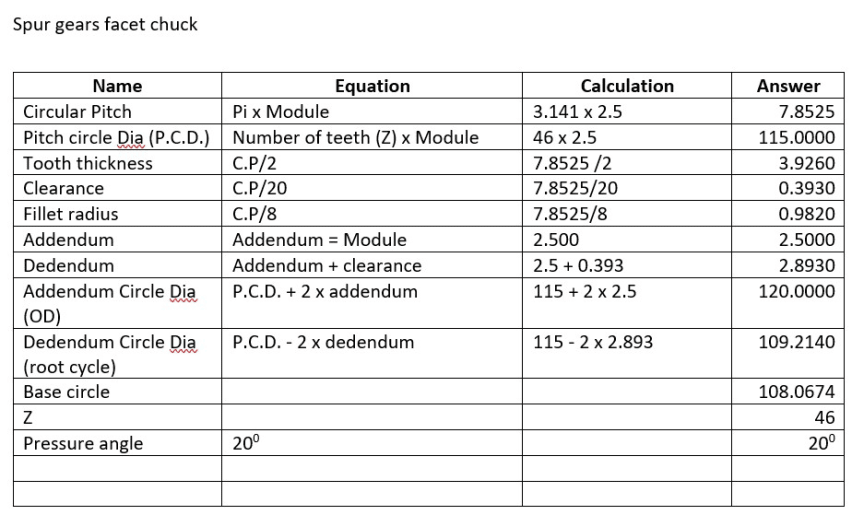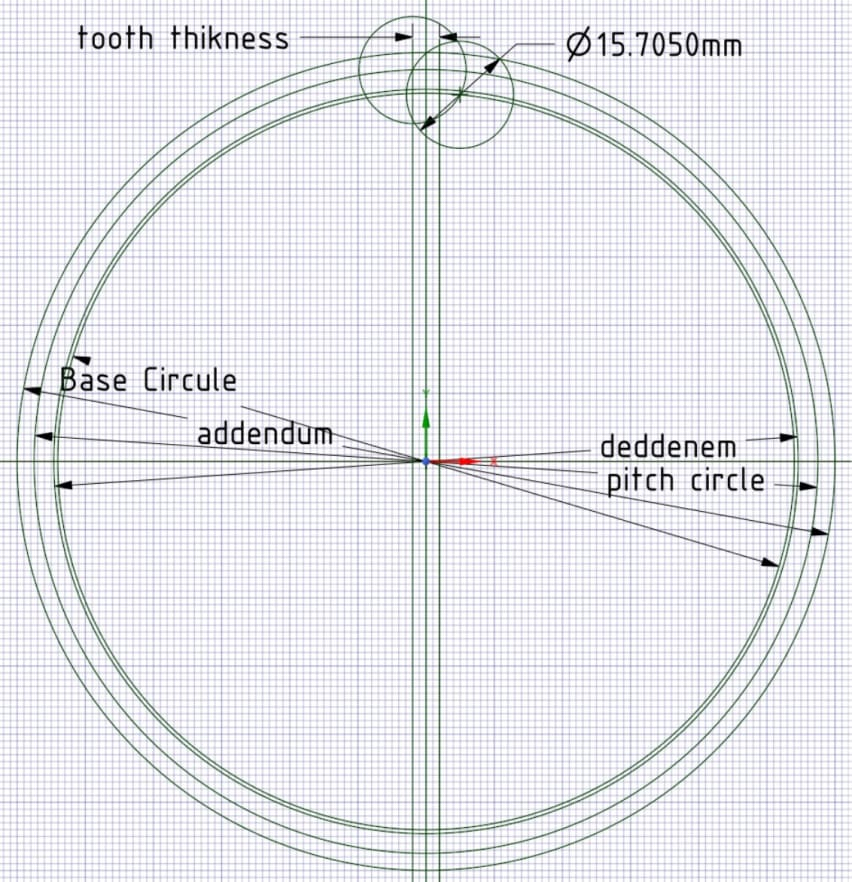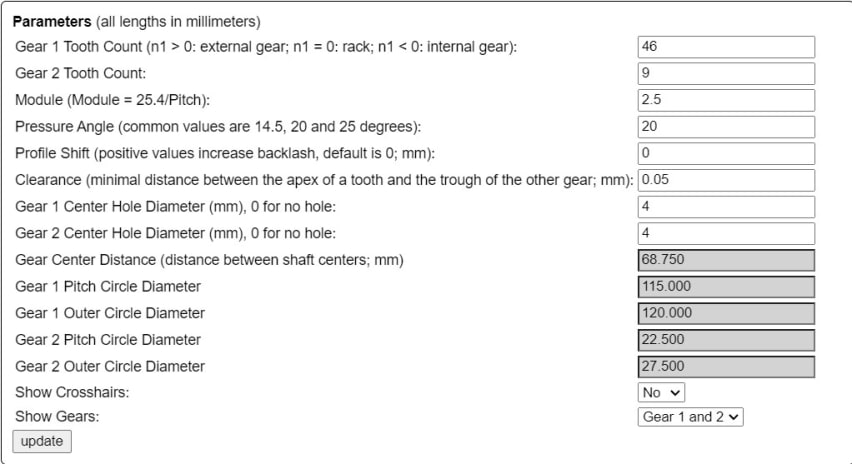# design spur gears using pressure angle

I used a calculator at https://evolventdesign.com/pages/calculators  to work out number of teeth, module and OD of the gears so it would fit in the parameters of my printer.  I wanted to draw up the gears in Designsparks using the pressuer angle method. The problem is the Basse Circule is smaller than the Dedendum Circule.  This cases the tooth to be shorter than the Addendum Circule.  My calculations are listed below and so is the drawing.

Is there an error in my calculations or the application?This is the result from the calculator from the website mention above